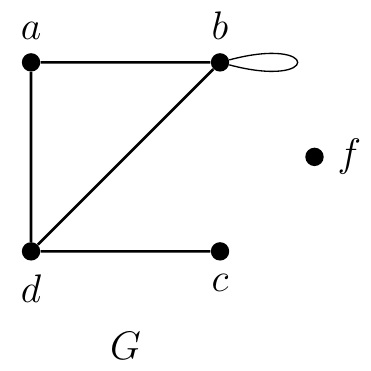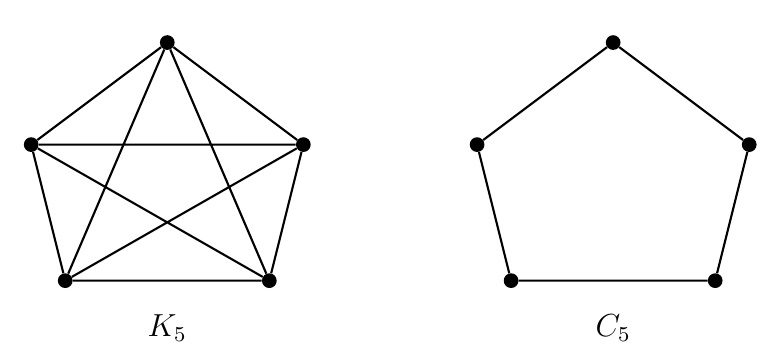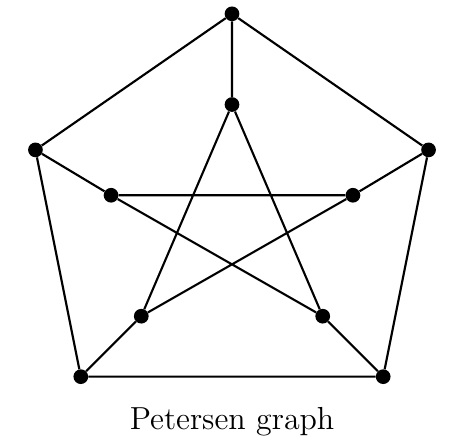Discrete Math Home

## Vertex Degrees

Definition (Degree). The degree of a vertex $$v$$ in a graph $$G$$ (not necessarily simple) is denoted by $$\operatorname{d}(v)$$ or $$\operatorname{deg}(v)$$ and is defined to be the number of edges incident with $$v$$ where a loop at $$v$$ contributes $$2$$ to $$\operatorname{d}(v)$$.Example. In the preceding graph $$G$$, $\operatorname{d}(f)=0,\;\operatorname{d}(c)=1,\;\operatorname{d}(d)=3,\;\operatorname{d}(a)=2,\;\operatorname{d}(b)=4.$ Theorem (The handshaking lemma). The sum of degrees of a graph $$G=(V,E)$$ is twice the number of its edges, i.e., $\sum_{v\in V} \operatorname{d}(v)=2|E|.$

A loop at a vertex $$v$$ contributes two to $$\operatorname{d}(v)$$. A non-loop edge $$e=\{u,v\}$$ contributes one to each of $$\operatorname{d}(u)$$ and $$\operatorname{d}(v)$$. Thus each edge contributes two to the degree-sum which implies $\sum_{v\in V} \operatorname{d}(v)=2|E|.$

Corollary. The number of vertices of odd degree in a graph is even.

Consider a graph $$G=(V,E)$$. Suppose $$V_o$$ and $$V_e$$ are the sets of vertices of $$G$$ of odd degree and even degree respectively. Then $\sum_{v\in V} \operatorname{d}(v)=\sum_{v\in V_o} \operatorname{d}(v)+\sum_{v\in V_e} \operatorname{d}(v).$ Since $$\sum_{v\in V} \operatorname{d}(v)=2|E|$$ by the handshaking lemma, $\sum_{v\in V_o} \operatorname{d}(v)+\sum_{v\in V_e} \operatorname{d}(v)=2|E| \implies \sum_{v\in V_o} \operatorname{d}(v)=2|E|-\sum_{v\in V_e} \operatorname{d}(v).$ Since $$\operatorname{d}(v)$$ is even for all $$v\in V_e$$, $$\sum_{v\in V_e} \operatorname{d}(v)$$ is even and consequently so is $\sum_{v\in V_o} \operatorname{d}(v)=2|E|-\sum_{v\in V_e} \operatorname{d}(v).$ Since $$\sum_{v\in V_o} \operatorname{d}(v)$$ is even and $$\operatorname{d}(v)$$ is odd for all $$v\in V_o$$, $$|V_o|$$ is even.

Example. In the preceding graph $$G$$, $\sum_{v\in V} \operatorname{d}(v) =\operatorname{d}(f)+\operatorname{d}(c)+\operatorname{d}(d)+\operatorname{d}(a)+\operatorname{d}(b)=0+1+3+2+4=10$ which is twice the number of edges of $$G$$. Also note that $$G$$ has two vertices of odd degree, viz, $$c$$ and $$d$$.

Definition (Degree sequence). The degree sequence of a graph $$G$$ is the nonincreasing sequence of vertex degrees of $$G$$.

Example. The degree sequence of the preceding graph $$G$$ is $$(4,3,2,1,0)$$.

Remark. If $$(d_1,d_2,\ldots,d_n)$$ is the degree sequence of a graph $$G=(V,E)$$, then $$d_1\geq d_2\geq \cdots \geq d_n$$, $$|V|=n$$, and $|E|=\frac{d_1+d_2+ \cdots +d_n}{2}.$

Example.

1. Is there any graph with the degree sequence $$(4,3,2,0)$$?

2. If possible, draw a graph with the degree sequence $$(4,2,2,0)$$.

Theorem (Erdös-Gallai theorem, 1960). $$(d_1,d_2,\ldots,d_n)$$ is the degree sequence of a simple graph if and only $$\sum_{i=1}^n d_i$$ is even and for all $$k=1,2,\ldots,n$$, $\sum_{i=1}^k d_i \leq k(k-1)+\sum_{i=k+1}^n \min\{d_i,k\}.$

Definition (Regular graph). A graph $$G$$ is $$k$$-regular if $$\operatorname{d}(v)=k$$ for all $$v\in V(G)$$.Example.

1. $$K_n$$, the complete graph on $$n$$ vertices, is a simple graph in which any two edges are adjacent. Note that $$K_n$$ is $$(n-1)$$-regular and $$|E(K_n)|=\binom{n}{2}=\frac{n^2-n}{2}$$.

2. $$C_n$$, the cycle on $$n\geq 3$$ vertices, is a simple graph whose vertices can be labeled as $$1,2,\ldots,n$$ so that $$E(C_n)=\{\{1,2\},\;\{2,3\},\ldots,\{n-1,n\},\;\{n,1\}\}$$. Note that $$C_n$$ is $$2$$-regular and $$|E(C_n)|=n$$.

$$P_n$$, the path on $$n\geq 3$$ vertices, is a simple graph which can be obtained from some $$C_n$$ by deleting one edge. Note that $$P_2=K_2$$ and $$P_1=K_1$$ which are the only regular paths.

3. $$W_n$$, the wheel on $$n\geq 4$$ vertices, is a simple graph obtained by joining a vertex to all the vertices of $$C_{n-1}$$. Note that $$W_4$$ is $$3$$-regular but $$W_n$$ is not regular for all $$n\geq 5$$.

4. Petersen graph is a famous graph on $$10$$ vertices which is $$3$$-regular.Problem. The complement of a simple graph $$G$$, denoted by $$\overline{G}$$ or $$G^c$$, is the simple graph with the same vertices as $$G$$ in which $$\{i,j\}$$ is an edge if and only if $$\{i,j\}$$ is not an edge in $$G$$. Suppose $$(d_1,d_2,\ldots,d_n)$$ is the degree sequence of a simple graph $$G$$.

1. Find the degree sequence of $$\overline{G}$$.

2. Use the degree sequence of $$\overline{G}$$, to write the number of edges of $$\overline{G}$$ in terms of $$n$$ and $$m$$, the number of edges of $$G$$.

Last edited